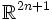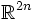# Whitney embedding theorem

View a complete list of embedding theorems
This fact is an application of the following pivotal fact/result/idea: Sard's theorem
View other applications of Sard's theorem OR Read a survey article on applying Sard's theorem
This fact is an application of the following pivotal fact/result/idea: existence of smooth partitions of unity
View other applications of existence of smooth partitions of unity OR Read a survey article on applying existence of smooth partitions of unity

## Statement

The Whitney embedding theorem states the following:

• Any compact connected differential manifold of dimension$n$, can be embedded inside$\R^{2n +1}$
• Any compact connected differential manifold of dimension$n$, can be immersed inside$\R^{2n}$

## Proof

### Proof ingredients

Two ingredients are used in the proof:

• Sard's theorem, or rather, the following corollary of Sard's theorem: if$m < n$, the image of any$m$-dimensional manifold in a$n$-dimensional manifold via a differentiable map, has measure zero in the latter.

We can use Sard's theorem to predict certain properties of maps that we construct.